# 点云配准各种方法总结[不定时更新]

本文主要用来记录我在学习过程中接触到的一些或新或旧的点云配准的方法，有些方法我可能还不怎么理解，如果有错误欢迎大家指出。

2018/04/15 添加LORAX
2018/04/08 添加DO法和结合法
2018/04/01 初版

我们都知道点云配准分为粗配准（Coarse Registration）和精配准（Fine Registration）两个阶段，本文同样也分为粗配准和精配准两个部分来说明。

## 粗配准方案

### LORAX

这是一篇采用机器学习的点云配准的论文，简单来说就是通过将点云用一个个球体分成很多个小快，对每一块投影成深度图，然后采用深度神经网络对深度图进行特征压缩，最后压缩成一个5×2的矩阵做为一个特征，也就是我们所说的描述子（descriptor）。通过这些描述子的位置关系可以进行粗配准，最后文章也还是采用了ICP进行精配准。原文链接：3D Point Cloud Registration for Localization Using a Deep Neural Network Auto-Encoder。具体的内容我单独写了一篇博客：阅读笔记

### 4点法（4-Points Congruent Sets，4PCS）

4点法由D Aiger，NJ Mitra，D Cohen-Or${}^{\left[1\right]}$$^{}$于2008年提出的一种快速地，鲁棒地的3D点云粗配准方法，原文链接见 4-points Congruent Sets for Robust Surface Registration

### Super 4PCS(Super 4-Points Congruent Sets)

Super 4PCS是由Nicolas Mellado，Dror Aiger，Niloy J. Mitra${}^{\left[2\right]}$$^{}$等人与2014年提出的一种对4PCS的加速方案。原文连接见Super4PCS: Fast Global Pointcloud Registration via Smart Indexing
网上很少能找到关于这篇文章的原理，我个人的理解是首先它通过记录点集$P$$P$$ab$$ab$,$cd$$cd$两条直线相交的角度$\theta$$\theta$，在点集$Q$$Q$中寻找匹配对时，额外计算两条直线相交的角度是否在$\theta$$\theta$的一定范围$\xi$$\xi$里，从而排除一些无效的匹配对来加速配准。
那么如何快速的找到角度在一定范围内的匹配对呢，Super4PCS采用的是类似与在球面上画圆的方法，如下图：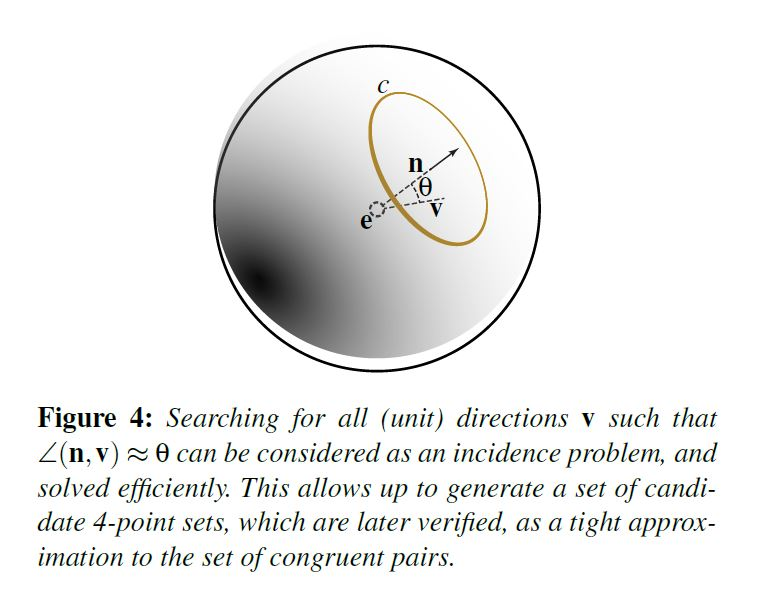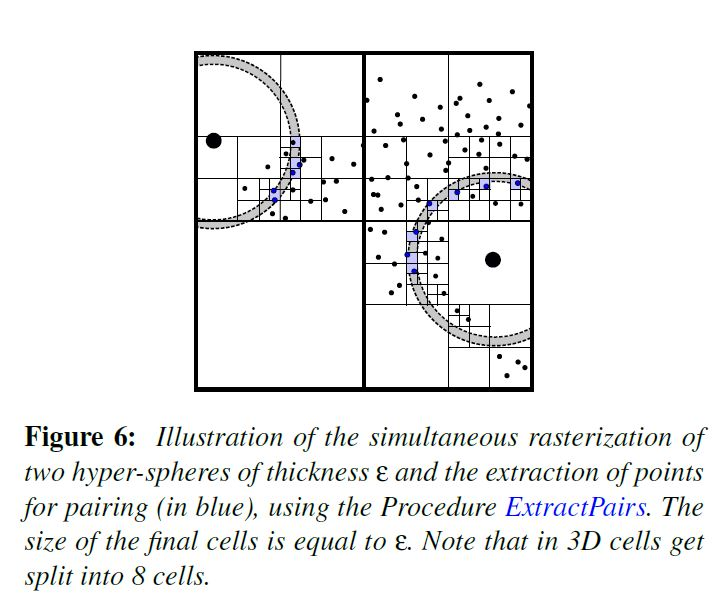### SK-4PCS（Semantic Keypoint 4-Points Congruent Sets）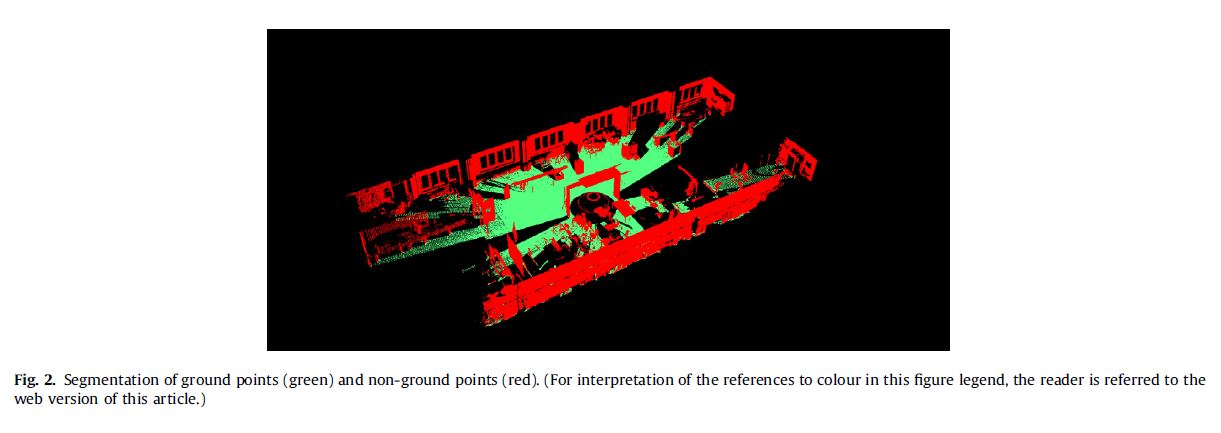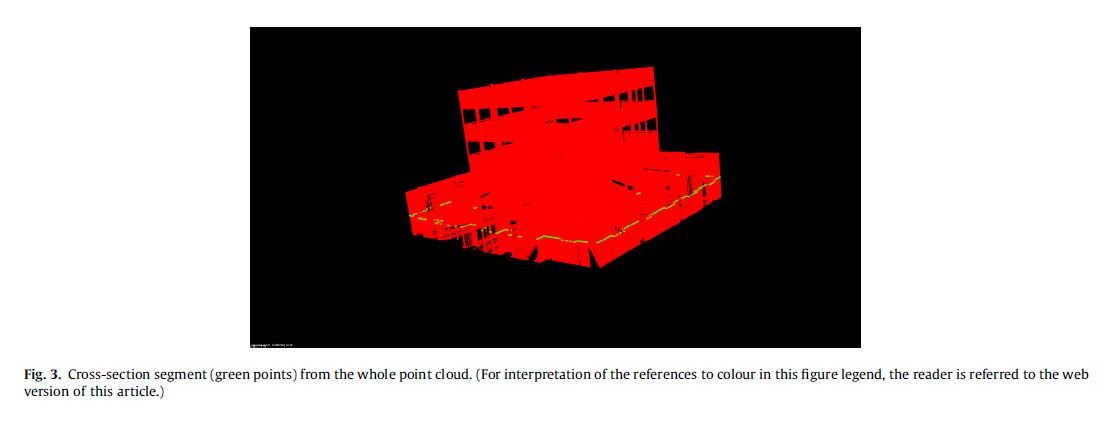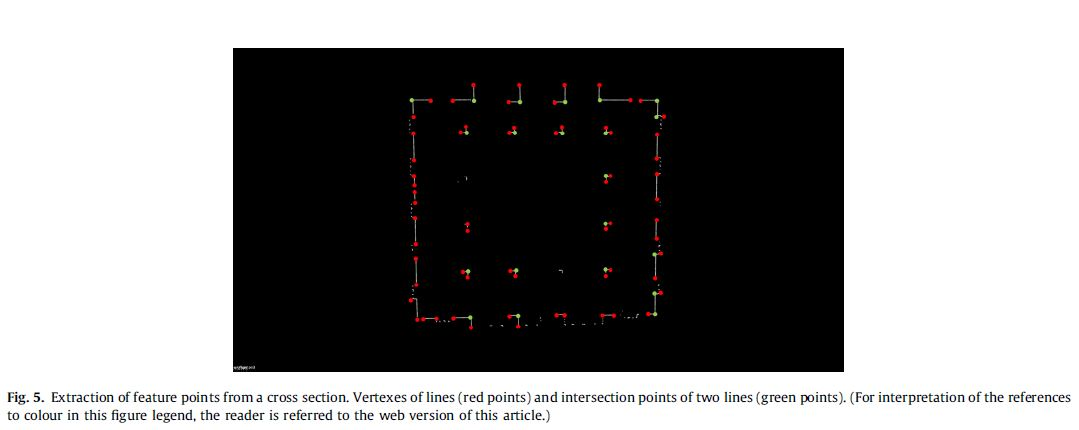在提取了每个横截面中的特征点后，我们需要对它们进行区分和连接，根据他们自身的位置，以及潜在位置赋予不通的标签。最终建立$target$$target$$source$$source$中语义点的意义对应关系。连接后的语义点如下图，其中蓝色线条连接特征点，红色点代表线段的顶点，绿色点代表线段的相交点，紫色三角形则是我们所定义的语义点，是连接线段与参考面的交点，存储着整条线段的语义信息（特征点的个数，每个特征点的标签）。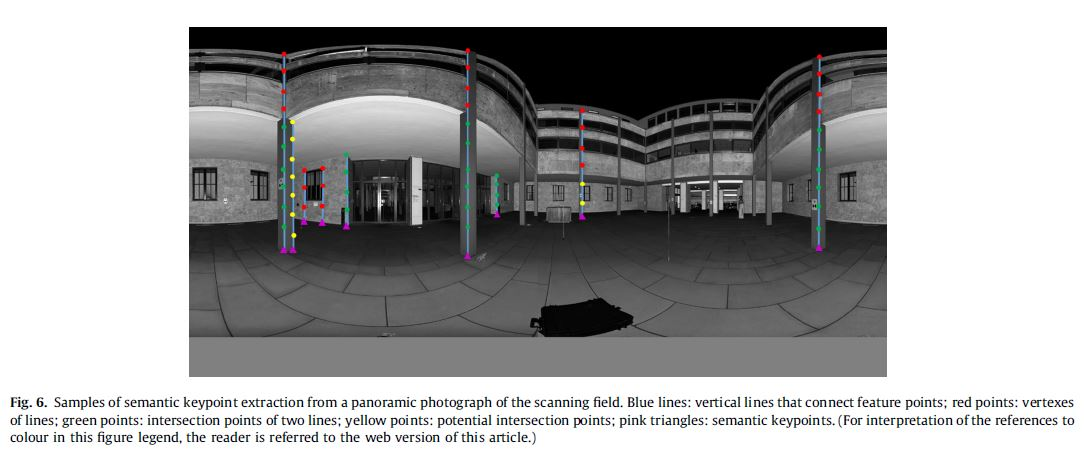### G-4PCS（Generalized 4-points congruent sets）

G-4PCS是由M Mohamad ， D Rappaport ， M Greenspan 提出的对4PCS的一种拓展，使4点法不在局限于共面的4点对。原文连接见：Super Generalized 4PCS for 3D Registration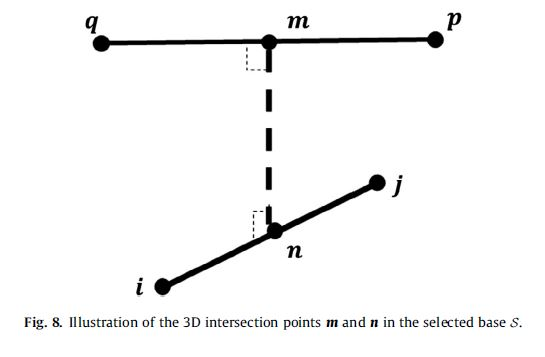${r}_{1}=\frac{||q-m||}{||q-p||}$

${r}_{2}=\frac{||i-n||}{||i-j||}$

${d}_{3}=||m-n||$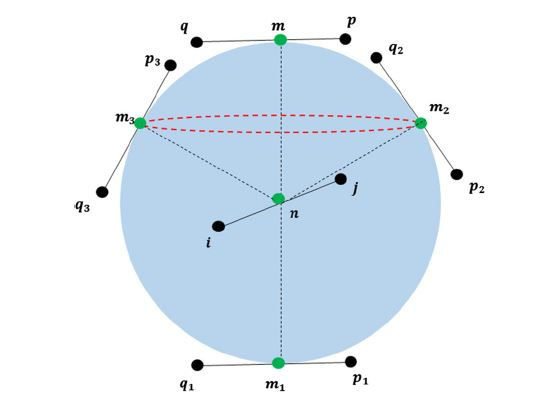我们可以找到很多满足上式的匹配对，例如：${\mathrm{\Gamma }}_{1}=\left\{i,j,{p}_{1},{q}_{1}\right\}$$\Gamma_1=\{i,j,p_1,q_1\}$${\mathrm{\Gamma }}_{2}=\left\{i,j,{p}_{2},{q}_{2}\right\}$$\Gamma_2=\{i,j,p_2,q_2\}$${\mathrm{\Gamma }}_{1}=\left\{i,j,{p}_{3},{q}_{3}\right\}$$\Gamma_1=\{i,j,p_3,q_3\}$，分别相交于$n,{m}_{1}$${n,m_1}$$n,{m}_{2}$${n,m_2}$$n,{m}_{3}$${n,m_3}$。因此，有必要在寻找匹配对时删去这些模糊的匹配对。在SK-4PCS中，作者对这种问题进行了分类，将其分为垂直类型和平面类型的两种模糊。
对于垂直类型的模糊，例如$\mathrm{\Gamma }$$\Gamma$${\mathrm{\Gamma }}_{1}$$\Gamma_1$，我们可以计算向量$\stackrel{\to }{nm}$$\overrightarrow {nm}$和向量$\stackrel{\to }{n{m}_{1}}$$\overrightarrow {nm_1}$,并和参考点云的向量做比较从而区分他们。
对于平面类型的模糊，如下图，我们定义一个通过向量$\stackrel{\to }{ij}$$\overrightarrow {ij}$来定义一个参考方向，并计算方位角${\alpha }_{2}$$\alpha_2$${\alpha }_{3}$$\alpha_3$从而进行区分。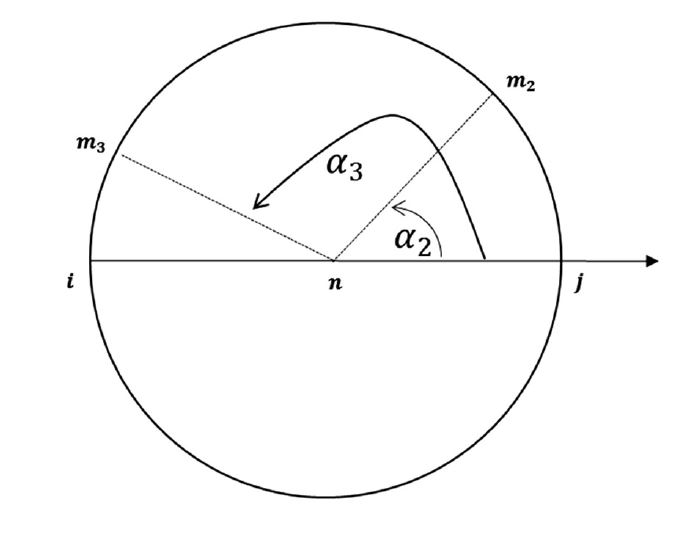## 精配准方案

精配准中，最经典最常用的方法就是迭代最近点法（Iterative Closest Point，ICP），而后的大多数方法都是ICP的变种。下文会简单介绍ICP算法的原理以及我在查阅论文中遇到的一些引用数较多的文章中所提到的算法。如果大家有什么经典或者新颖的算法也可以留言大家共同讨论交流，学习进步。

04/08/2018

### DO（Discriminative Optimization）

与传统的构建损失函数，求解损失函数不同，　这篇文章提出了DO（Discriminative Optimization）的方法，通过学习更新序列来解决一系列计算机视觉上的问题。原文见2017年CVPR：Discriminative Optimization: Theory and Applications to Point Cloud Registration${}^{\left[11\right]}$$^{}$

#### 1. 更新法则

DO采用如下的更新法则：

$\begin{array}{}\text{(2)}& {X}_{k+1}={X}_{k}-{D}_{k+1}h\left({X}_{k}\right)\end{array}$

其中，$h:{\mathbb{R}}^{p}\to {\mathbb{R}}^{f}$$h:\Bbb R^p \rightarrow \Bbb R^f$为特征提取函数，矩阵${D}_{k+1}\in {\mathbb{R}}^{p×f}$$D_{k+1} \in \Bbb R^{p \times f}$将特征$h\left({X}_{k}\right)$$h(X_k)$转换为更新向量，矩阵${D}_{k+1}$$D_{k+1}$由训练数据得来。

#### 2. 训练方法

给定一个训练集${\left({X}_{0}^{\left(i\right)},{X}_{\ast }^{\left(i\right)},{h}^{\left(i\right)}\right)}_{i=1}^{N}$${(X_0^{(i)},X_*^{(i)},h^{(i)})}_{i=1}^N$，其中${X}_{0}^{\left(i\right)}\in {\mathbb{R}}^{p}$$X_0^{(i)} \in \Bbb R^p$是第$i$$i$个问题的实例，${X}_{\ast }^{\left(i\right)}\in {\mathbb{R}}^{p}$$X_*^{(i)} \in \Bbb R^p$为真实值（groundt ruth）,而${h}^{\left(i\right)}：{\mathbb{R}}^{p}\to {\mathbb{R}}^{f}$$h^{(i)}：\Bbb R^p \rightarrow \Bbb R^f$为第$i$$i$个实例的特征，DO的目标就是学习更新序列$\left\{{D}_{k}{\right\}}_{k}$$\{D_k\}_k$，使得${X}_{0}^{i}$$X_0^{i}$更新为${X}_{\ast }^{i}$$X_*^{i}$，学习规则采用如下最小二乘回归函数：

$\begin{array}{}\text{(2)}& {D}_{k+1}=\mathrm{arg}\underset{\stackrel{~}{D}}{min}\frac{1}{N}\sum ||{X}_{\ast }^{\left(i\right)}-{X}_{k}^{\left(i\right)}+\stackrel{~}{D}{h}^{\left(i\right)}\left({X}_{k}^{\left(i\right)}\right)|{|}^{2}\end{array}$

在学习了${D}_{k+1}以后$$D_{k+1}以后$，可以用（1）来更新${X}_{k}^{\left(i\right)}$$X_k^{(i)}$，然后继续学习下一层，不断重复这个过程，直到满足终止条件。当${X}_{k}^{\left(i\right)}\approx {X}_{\ast }^{\left(i\right)}$$X_k^{(i)} \approx X_*^{(i)}$，式（3）会强制$\stackrel{~}{D}{h}^{\left(i\right)}\left({X}_{k}^{\left(i\right)}\right)$$\tilde D h^{(i)}(X_k^{(i)})$接近于0，也就是在${X}_{\ast }^{\left(i\right)}$$X_*^{(i)}$附近引入驻点。在实际中，为了防止过拟合，加入了ridge regression:

$\begin{array}{}\text{(3)}& \underset{\stackrel{~}{D}}{min}\frac{1}{N}\sum _{i=1}^{N}||{X}_{\ast }^{\left(i\right)}-{X}_{k}^{\left(i\right)}+\stackrel{~}{D}{h}^{\left(i\right)}\left({X}_{k}^{\left(i\right)}\right)|{|}^{2}+\frac{\lambda }{2}||\stackrel{~}{D}|{|}_{F}^{2}\end{array}$

#### 3. 配准应用

设$M\in {\mathbb{R}}^{3×{N}_{M}}$$M \in \Bbb R^{3 \times N_M}$为模型点云矩阵，$S\in {\mathbb{R}}^{3×{N}_{S}}$$S \in \Bbb R^{3 \times N_S}$为场景模型矩阵。我们的目标是找到$S\to M$$S \rightarrow M$的最佳变化矩阵。
由于大部分感兴趣的点云都是由物体表面组成的，文中提出了一种基于统计场景中的点在模型点的前方还是后方的特征函数用于矩阵学习，如下图：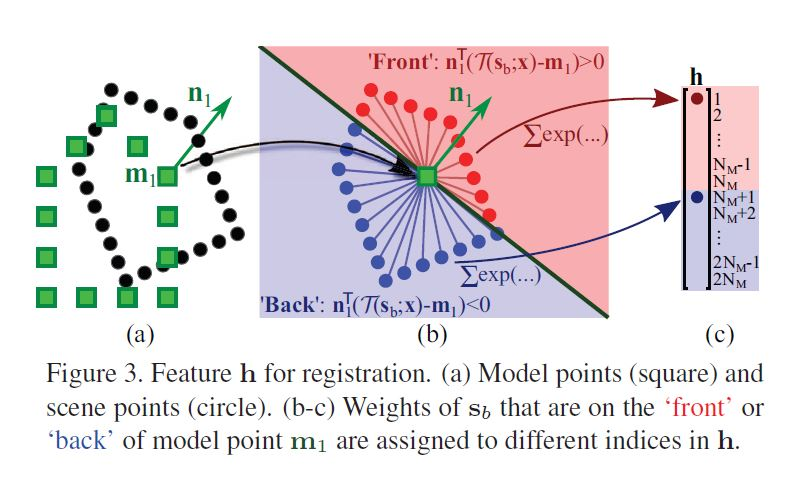我们定义${\stackrel{\to }{n}}_{a}\in {\mathbb{R}}^{3}$$\vec n_a \in \Bbb R^3$为模型点${m}_{a}$$m_a$由其领域计算得来的法向量。$\mathrm{\Gamma }\left(y;x\right)$$\Gamma(y;x)$使用参数$x$$x$$y$$y$做刚性变换。${m}_{a}$$m_a$的前方点定义为：${S}_{a}^{+}=\left\{{s}_{b}:{\stackrel{\to }{n}}_{a}^{\mathrm{\top }}\left(\mathrm{\Gamma }\left({s}_{b};x\right)-{m}_{a}\right)>0\right\}$$S_a^+=\{s_b:\vec n_a^{\top}(\Gamma(s_b;x)-m_a) \gt 0\}$${S}_{a}^{-}$$S_a^-$则包含其他的场景点，对于$h:{\mathbb{R}}^{6}×{\mathbb{R}}^{3}\to {\mathbb{R}}^{2{N}_{M}}$$h:\Bbb R^6 \times \Bbb R^{3} \rightarrow \Bbb R^{2N_M}$定义如下：

$\begin{array}{}\text{(4)}& \left[h\left(x;S\right){\right]}_{a}=\frac{1}{z}\sum _{{s}_{b}\in {S}_{a}^{+}}exp\left(-\frac{1}{{\sigma }^{2}}||\mathrm{\Gamma }\left({s}_{b};x\right)-{m}_{a}|{|}^{2}\right)\end{array}$

$\begin{array}{}\text{(5)}& \left[h\left(x;S\right){\right]}_{a+{N}_{M}}=\frac{1}{z}\sum _{{s}_{b}\in {S}_{a}^{-}}exp\left(-\frac{1}{{\sigma }^{2}}||\mathrm{\Gamma }\left({s}_{b};x\right)-{m}_{a}|{|}^{2}\right)\end{array}$

为了加速算法，输入数据会被归一化到$\left[-1,1\right]$$[-1,1]$，训练数据来自对模型的随机采样，并进行随机变换做为ground truth进行训练。

### 结合法

结合法是将点云的颜色信息与几何信息相结合经行配准，原文见2017年ICCV：Colored Point Cloud Registration Revisited${}^{\left[10\right]}$$^{}$。文中回顾了RGB-D深度图像基于颜色的配准方案和基于几何位置的配准的方案，并将两者的相结合，并推广至无序点云。

#### 1. RGB-D

一副RGB-D图像由颜色信息$I$$I$和深度信息$D$$D$组成，这里我们采用灰度信息来简化问题。给定一对RGB-D图像$\left({I}_{i},{D}_{i}\right)$$(I_i,D_i)$$\left({I}_{j},{D}_{j}\right)$$(I_j,D_j)$以及一个粗略的初始矩阵${T}^{0}$$T^0$，我们的目标是找到一个使两幅图像紧密配准的最佳变换矩阵。
基于颜色信息的目标函数${E}_{I}$$E_I$为：

${E}_{I}\left(T\right)=\sum \left({I}_{i}\left({x}^{{}^{\prime }}\right)-{I}_{j}\left(x\right){\right)}^{2}$

其中，$x=\left(u,v{\right)}^{T}$$x = (u,v)^T$是RGB-D图像$\left({I}_{j},{D}_{j}\right)$$(I_j,D_j)$中的一个像素，而${x}^{{}^{\prime }}=\left({u}^{{}^{\prime }},{v}^{{}^{\prime }}{\right)}^{T}$$x^{'} = (u^{'},v^{'})^T$是点$x$$x$在图像$\left({I}_{i},{D}_{i}\right)$$(I_i,D_i)$中的对应点。这种对应关系是通过将深度像素$\left(x,{D}_{j}\left(x\right)\right)$$(x,D_j(x))$$\left({I}_{j},{D}_{j}\right)$$(I_j,D_j)$的空间中转换为3D点，并将其变换$T$$T$后投影到图像$\left({I}_{i},{D}_{i}\right)$$(I_i,D_i)$中建立的，具体如下：

${x}^{{}^{\prime }}={g}_{uv}\left(s\left(h\left(x,{D}_{j}\left(x\right)\right),T\right)\right)$

其中，$h$$h$是将深度像素转换为3D点，$s$$s$为刚性变换，$g$$g$$h$$h$的逆函数，将3D点转换为深度像素。
同样的，我们根据深度信息也可以如此定义目标函数${E}_{D}$$E_D$，由两幅点云是在不同的相机空间中测量得到的，直接比较深度值会导致一个错误的结果， 所以这里我们采用弯曲深度。

${E}_{D}\left(\right)T=\sum _{x}\left({D}_{i}\left({x}^{{}^{\prime }}\right)-{g}_{d}\left(s\left(h\left(x,{D}_{j}\left(x\right)\right),T\right)\right){\right)}^{2}$

如此我们可以通过下式$E$$E$，将两者进行结合，其中$\sigma \in \left[0,1\right]$$\sigma \in [0,1]$为一常数，用于平衡两者间的关系。

$E\left(T\right)=\left(1-\sigma \right){E}_{I}\left(T\right)+\sigma {E}_{D}\left(T\right)$

#### 2. Color Point Cloud

接着，文章将上述方法拓展至普通点云。$P$$P$为一有色点云，$C\left(p\right)$$C(p)$为颜色检索函数，我们需要将颜色建设函数转变为一个连续函数${C}_{p}\left(u\right)$$C_p(u)$以便使用其梯度。
因此，文中为每一个点$p\in P$$p \in P$引入了虚拟正交相机这一概念，其沿着${\stackrel{\to }{n}}_{p}$$\vec n_p$，观察点$p$$p$，虚拟相机的观察面为点$p$$p$的切平面，设$\stackrel{\to }{u}$$\vec u$是从点$p$$p$开始的位于切平面上的一个向量，$\stackrel{\to }{u}\cdot {\stackrel{\to }{n}}_{p}=0$$\vec u \cdot \vec n_p =0$，则函数${C}_{p}\left(u\right)$$C_p(u)$可一阶近似为：

${C}_{p}\left(u\right)\approx C\left(p\right)+{d}_{p}\mathrm{\top }u$

其中${d}_{p}$$d_p$是函数$\left({C}_{p}\left(u\right)\right)$$(C_p(u))$的梯度，由对其临近点进行最小二乘拟合得来。我们定义$f\left(s\right)$$f(s)$为投影3D点到切平面的函数：

$f\left(s\right)=s-{\stackrel{\to }{n}}_{p}\left(s-p{\right)}^{\mathrm{\top }}{\stackrel{\to }{n}}_{p}$

同样地我们假设虚拟相机有着深度信息因此定义连续深度函数${G}_{p}\left(u\right)$$G_p(u)$，他的原点的梯度为0，${0}_{p}$$0_p$为相机原点，则起一阶近似为：

${G}_{p}\left(u\right)\approx \left({o}_{p}-P{\right)}^{\mathrm{\top }}{\stackrel{\to }{n}}_{p}$

这样，对于两个点云$P,Q$$P,Q$我们可以定义联合最优函数$E\left(T\right)$$E(T)$:

$E\left(T\right)=\left(1-\sigma \right){E}_{C}\left(T\right)+\sigma {E}_{G}\left(t\right)$

令$p,q$$p,q$为每次迭代后$P$$P$${T}^{k}Q$$T^kQ$的对应点集$K=\left\{\left(p,q\right)\right\}$$K=\{(p,q)\}$${q}^{{}^{\prime }}$$q^{'}$为点$q$$q$在点$p$$p$的切平面上的投影

${q}^{{}^{\prime }}=f\left(s\left(q,T\right)\right)9$

则${E}_{C}，{E}_{G}$$E_C，E_G$分别如下定义：

${E}_{C}\left(T\right)=\sum _{\left(p,q\right)\in K}\left({C}_{p}\left({q}^{{}^{\prime }}\right)-C\left(q\right)\right)$

如何求解，可以参考原文。同时为了避免陷入局部最优，文中采用不同大小的体素滤波器对原始点云进行滤波产生了不同精度的点云金字塔，通过不断提高输入的点云密度使得配准精度由粗略转为精细。

04/01/2018

### ICP（Iterative Closest Point）

ICP算法是由PJ Besl ， ND Mckay ${}^{\left[6\right]}$$^{}$在1992年提出的一种高层次的基于自由形态曲面的配准方法。原文连接见：A method for registration of 3-D shapes。简单来说就是不停的循环，在两个点云间找到最近的对应点，由这些对应点计算出一个使按照此旋转平移矩阵变换后对应点距离最小的RT矩阵，并进行变换，重复以上步骤，直到某些值达到设定的阈值则停止迭代。对于点集$P,Q$$P,Q$，算法步骤如下：

• 计算点集${Q}_{i}$$Q_i$中的若干点在点集$P$$P$中的最近点
• 求上述对应最近点对平均距离最小的旋转变化矩阵$R{T}_{i}$$RT_i$
• 对点集${Q}_{i}$$Q_i$使用上述求的$R{T}_{i}$$RT_i$进行变换得到点集${Q}_{i+1}$$Q_{i+1}$
• 若点集$P$$P$与点集${Q}_{i+1}$$Q_{i+1}$的某些目标函数小于一定阈值（例如最近点的平均距离），则可以停止迭代

### 模型对应法

好吧，这个名字是我起的。文章并没有给这个方法起一个名字。这篇文章是我在查阅资料时遇到的一篇100+引用的文章，原文是由T Rabbani ， S Dijkman ， FVD Heuvel ， G Vosselman 发表在ISPRS上的${}^{\left[7\right]}$$^{}$。原文连接见：An integrated approach for modelling and global registration of point clouds。这篇文章有趣之处在于提出了一种对点云进行建模与配准同时进行的方法。原文中有大量的公式推导，这里不会进行赘述。文章中针对工业环境存在大量的简单几何体例如平面，圆柱，圆环等实际提出两种方法，一种间接法，一种直接法。其中间接法是将物体拟合和场景匹配分开来做，而直接法则是同时决定物体的形状和位置参数以及配准参数。

#### 1. 间接法

• 在不通的场景中为每个点赋予一个标记，将其标记在一个具体的物体上，文中采用Hoover, A., Jean-Baptiste, G. et al.在1996年提出的方案${}^{\left[8\right]}$$^{}$An experimental comparison of range image segmentation algorithms
• 在每个场景中，对每一个物体进行基于最小均方差的参数拟合
• 将物体参数做为输入，使用最小均方差法去计算最后的变换矩阵的参数，这一步将物体参数的均方差做为场景的变换参数的目标函数使其最小

$\underset{\left\{R\right\},\left\{t\right\}}{min}\sum _{i=1}^{C}\sum _{j=1}^{{M}^{i}}{\mathrm{\Delta }}_{{u}_{{i}_{j}}}^{2}$

${\mathrm{\Delta }}_{{u}_{{i}_{j}}}={\mathrm{\Psi }}_{{R}_{i}^{a}{t}_{i}^{a}}\left({u}_{{i}_{j}}^{a}\right)-{\mathrm{\Psi }}_{{R}_{i}^{b}{t}_{i}^{b}}\left({u}_{{i}_{j}}^{b}\right)$

在等式中，$C$$C$是指存在的对应关系的个数，每一个对应关系都包含有两个物体$a，b$$a，b$。第$i$$i$个对应关系的模型由${M}_{i}$$M_i$个拟合参数。${\mathrm{\Psi }}_{{R}_{i}^{a}{t}_{i}^{a}}$$\Psi_{R^a_i t^a_i}$表示对场景使用变换对${R}_{i}^{a}{t}_{i}^{a}$$R^a_i t^a_i$进行变换。物体$a$$a$由它的参数${u}_{{i}_{j}}^{a}$$u^a_{i_j}$建模而成。我们的目标是使得存在对应关系的物体对的参数的均方差${\mathrm{\Delta }}_{{u}_{{i}_{j}}}^{2}$$\Delta^{2}_{u_{i_j}}$最小。因此，我们可以调整参数$\left\{R\right\}$$\{R\}$$\left\{t\right\}$$\{t\}$。那么如何求解这个最值问题，具体可以参考原文的推导。

#### 2. 直接法

• 配准参数预估，结果仅仅是对配准参数的预估
• 综合调整，配准参数和模型参数同时被估算

$\underset{\left\{R\right\},\left\{t\right\}}{min}\sum _{i=1}^{O}\sum _{j=1}^{{P}^{i}}{\mathrm{\Omega }}_{i}^{2}\left({R}_{i}{p}_{j}+{t}_{i}\right)$

 Homepage B T. 4-points Congruent Sets for Robust Surface Registration[J].

 Mellado N, Aiger D, Mitra N J. Super 4PCS Fast Global Pointcloud Registration via Smart Indexing[J]. Computer Graphics Forum, 2015, 33(5):205-215.

 Ge X. Automatic markerless registration of point clouds with semantic-keypoint-based 4-points congruent sets[J]. Isprs Journal of Photogrammetry & Remote Sensing, 2017, 130:344-357.

 Theiler P W, Wegner J D, Schindler K. Keypoint-based 4-Points Congruent Sets – Automated marker-less registration of laser scans[J]. Isprs Journal of Photogrammetry & Remote Sensing, 2014, 96(11):149-163.

 Mohamad M, Ahmed M T, Rappaport D, et al. Super Generalized 4PCS for 3D Registration[C]// International Conference on 3d Vision. IEEE Computer Society, 2015:598-606.

 Besl P J, Mckay N D. A Method for Registration of 3-D Shapes[M]. IEEE Computer Society, 1992.

 Rabbani T, Dijkman S, Heuvel F V D, et al. An integrated approach for modelling and global registration of point clouds[J]. Isprs Journal of Photogrammetry & Remote Sensing, 2007, 61(6):355-370.

 Hoover A, Jean-Baptiste G, Jiang X, et al. An experimental comparison of range image segmentation algorithms[J]. IEEE Trnasactions on Pattern Analysis & Machine Intelligence, 1996, 18(7):673-689.

 Atkinson K B. Close Range Photogrammetry and Machine Vision[J]. Empire Survey Review, 2001, 34(266):276-276.

 Park J, Zhou Q Y, Koltun V. Colored Point Cloud Registration Revisited[C]// IEEE International Conference on Computer Vision. IEEE Computer Society, 2017:143-152.

 Vongkulbhisal J, Torre F D L, Costeira J P. Discriminative Optimization: Theory and Applications to Point Cloud Registration[C]// IEEE Conference on Computer Vision and Pattern Recognition. IEEE, 2017:3975-3983.01-07

#### 点云完全配准算法比较

03-05

03-051万+

#### 点云配准——（1）几种点云配准算法比较

11-117141

#### 点云的粗配准和精配准

07-193879

#### 3D点云深度学习PointNet源码解析——数据预处理

09-1613万+

#### 从入门到精通，Java学习路线导航（附学习资源）

03-31262

#### 点云配准各种方法总结

01-091万+

#### 三维计算机视觉（八）--点云配准

09-12351

#### PCL点云配准（3）

06-071万+

#### 点云配准算法的综述

01-028万+

#### 20道你必须要背会的微服务面试题，面试一定会被问到

10-23373

#### 最新微信小程序源码

03-264万+

#### 微信小程序源码-合集1

04-211116

#### 三维点云配准的方法总结

03-051086

#### 点云配准(CloudCompare软件)

03-112万+

#### 吹爆了！程序员自学Python，不如9岁小孩，网友：我不信....

04-216338

#### 【PCL学习】点云配准——ICP

05-103390

#### 点云配准

08-261万+

#### PCL：ICP点云配准

04-201万+

#### 三维点云配准

04-141358

#### 点云配准——（2）四点法

08-111365

#### 把点云（点集）里面的点全部标准化到-1到1区间内

06-281729

#### 点云配准ICP算法详解

07-076250

#### 点云配准算法之super-4pcs

03-253万+

#### 当HR压你价，说你只值7K，你该怎么回答？

01-105万+

#### 讲真，这两个IDE插件，可以让你写出质量杠杠的代码

03-243万+

#### 为什么程序员做外包会被瞧不起？

04-092万+

#### 代码注释如此沙雕，会玩还是你们程序员！

03-1610万+

#### 我说我不会算法，阿里把我挂了。

03-203万+

#### 【Java面试题】List如何一边遍历，一边删除？

03-1210万+

#### 如果你是老板，你会不会踢了这样的员工？

03-0413万+

#### 和黑客斗争的 6 天！

03-012万+

#### 将一个接口响应时间从2s优化到 200ms以内的一个案例

05-141万+

#### 用python打开电脑摄像头，并把图像传回qq邮箱【Pyinstaller打包】

03-30503

#### 教程：Python Open3d 完成 ICP 点云配准

04-256万+

#### 总结了 150 余个神奇网站，你不来瞅瞅吗？

03-224万+

#### 男生更看重女生的身材脸蛋，还是思想？

03-231万+

#### 曾经废寝忘食学到的技术，现在都没用了......

04-075万+

#### 我说我懂多线程，面试官立马给我发了offer

03-263万+

#### 终于懂了TCP和UDP协议区别

05-173605

#### 你不知道的SVD 算法------点云配准+绝对定向+坐标转换

03-087万+

#### 女程序员，为什么比男程序员少？？？

03-304万+

#### Python爬虫，高清美图我全都要（彼岸桌面壁纸）

03-1311万+

#### 我入职阿里后，才知道原来简历这么写

03-195万+

#### !大部分程序员只会写3年代码

08-164078

#### 点集配准与ICP算法(一)

03-258万+

#### 面试阿里p7，被按在地上摩擦，鬼知道我经历了什么？

03-222万+

#### HTTP与HTTPS的区别

03-299万+

#### 大三实习生，字节跳动面经分享，已拿Offer

06-156975

#### 使用深度学习进行点云匹配（一）

03-234万+

#### 程序员毕业去大公司好还是小公司好？

04-303万+

#### 如何优雅的替换掉代码中的ifelse

02-274万+

#### 再不跳槽，应届毕业生拿的都比我多了！

02-151万+

#### 强烈推荐 10 款珍藏的 Chrome 浏览器插件

07-27369

#### 一分钟详解PCL中点云配准技术

03-082万+

#### 搜狗输入法也在挑战国人的智商！

09-14998

#### 点云配准算法和开源软件库

03-274万+

#### 无代码时代来临，程序员如何保住饭碗？

03-193万+

#### 2020阿里全球数学大赛：3万名高手、4道题、2天2夜未交卷

03-102万+

#### 刚回应！删库报复！一行代码蒸发数10亿！

03-055万+

#### 讲一个程序员如何副业月赚三万的真实故事

04-175万+

#### Java岗开发3年，公司临时抽查算法，离职后这几题我记一辈子

01-174万+

#### GitHub 上有哪些适合新手跟进的优质项目？

01-1411万+

#### 没用过这些 IDEA 插件？怪不得写代码头疼

05-084万+

#### 面试：第十六章：Java中级开发（16k）

01-092万+

#### 有网友说：2020年还不懂Spring就放弃Java吧？

03-234万+

#### 程序员为什么千万不要瞎努力？

01-307万+

#### 非典逼出了淘宝和京东，新冠病毒能够逼出什么？

04-067万+

#### 终于，月薪过5万了！

04-04307

#### 点云配准网络pointnetLK解读笔记（上）

06-264万+

#### PCL中的点云配准（Registration）ICP算法

02-111万+

#### 啥水平？谷歌程序员：我用东北方言编程

03-084万+

#### 上班一个月，后悔当初着急入职的选择了©️2019 CSDN 皮肤主题: 编程工作室 设计师: CSDN官方博客点击重新获取扫码支付